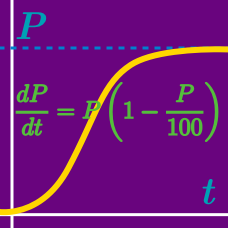Calculus

# Differential Equations - Variable Separable

What is the solution of the following differential equation: $\left(\frac{dy}{dx}\right)^{5}-x\frac{dy}{dx}+y=0?$

Note: $C$ is a constant.

What is the solution of the differential equation $\frac{1}{5}\left(\frac{1}{{x}^{3}}\frac{dx}{dy}-\frac{1}{{x}^{2}}y\right) =y\sin{{y}^{2}} ?$

Details and assumptions

Use $C$ as the constant of integration.

What is the solution of the differential equation \begin{aligned} (8x^2-5yx^2)\frac{dy}{dx}+(5y^3+xy^3) &=0, \\ y(1) &=1? \end{aligned}

What is the solution of the differential equation \begin{aligned} \log{\frac{dy}{dx}} &=8x+7y,\\ y(0)&=0? \end{aligned}

Note: In this problem, $\log(x)$ is the natural logarithm (log base e) of $x.$

What is the solution of the differential equation $\log{\frac{dy}{dx}}=5x+2y, y(0)=0?$

×